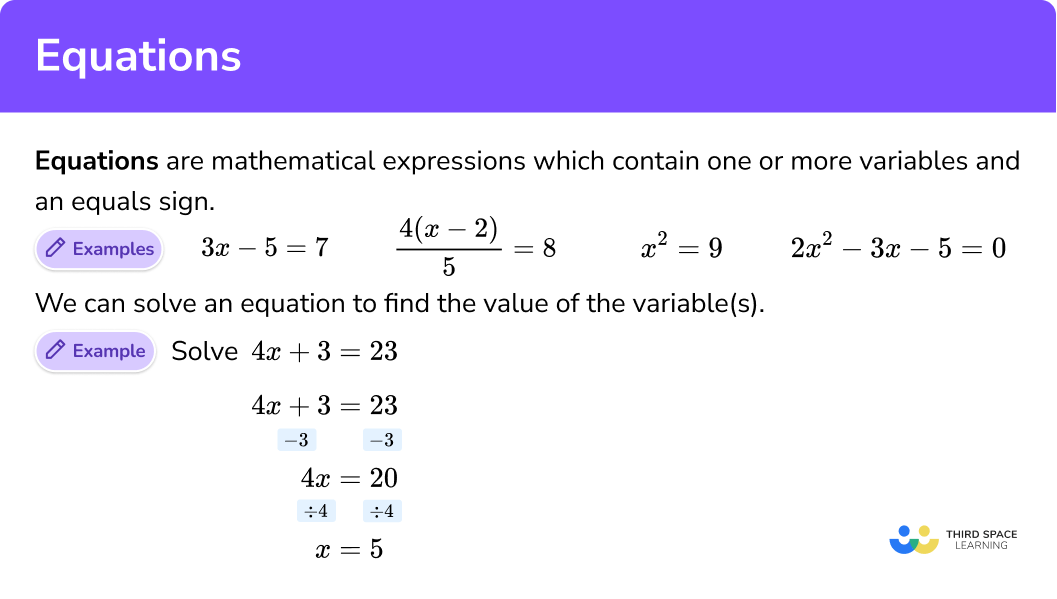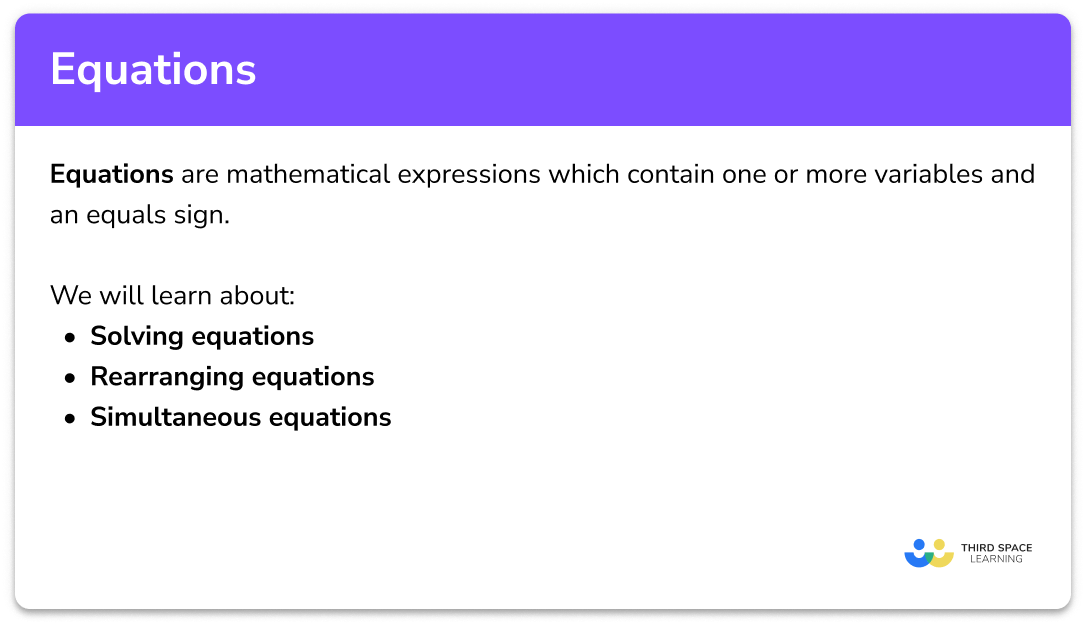GCSE Maths Algebra

Equations

# Equations

Here we will learn about equations, including solving equations, linear equations, quadratic equations, simultaneous equations and rearranging equations.

There are also equations worksheets based on Edexcel, AQA and OCR exam questions, along with further guidance on where to go next if you’re still stuck.

## What are equations?

Equations are mathematical expressions which contain a variable and an equals sign.

We can solve different types of equations to find the value of the variable.

E.g.

\begin{aligned} &3x-5=7 \\\\\\ &\frac{4(x-2)}{5}=8 \\\\\\ &x^2=9 \\\\\\ &2x^2-3x-5=0 \end{aligned}

### What are equations?### What are simultaneous equations?

Simultaneous equations are a pair of equations with two variables.  They can be solved to find a pair of values which make both equations true at the same time.

Step-by-step guide: Simultaneous equations

E.g. Linear simultaneous equations

\begin{aligned} x+y&=10\\\\ x-y&=4 \end{aligned}

y=x^2-6x+8

y=2x+1

### What is rearranging equations?

Rearranging equations means we change the subject of the equation to display it in a different way.

Step-by-step guide: Rearranging equations

E.g.

The subject of the following equation is currently y .

y=3x+2

We can rearrange the mathematical equation to make x the subject:

x=\frac{y-2}{3}

Sometimes an equation is a formula. This is when it is used to solve a specific problem.

E.g.

Here is the formula to work out the area of a circle:

A=\pi r^2

It could be rearranged to find the radius if we are given the area:

r=\sqrt{\frac{A}{\pi }}

### Equations and identities

Equations and identities look very similar, but are two different things.

An identity is true for all values of the variable, whereas the equation is true for only certain values.

For example, the equation:

3x+5=14

can be solved, so it is true only when x=3.

However the identity:

3(x+2)\equiv3x+6

It can not be solved. The left-hand side always equals the right-hand side for all values of x.

At A-level you will be introduced to a variety of trigonometric identities to enable you to prove trigonometric expressions such as each pythagorean identity (derived from the pythagoras theorem) or the double angle identities.

### Identity sign

Algebraic identities use the ‘≡’ symbol. It is like an equals sign =, but it means ‘identical to’.

### Iteration

Iteration is a process in which the approximate solution is determined using an iterative formula and a known starting number. When using iteration to calculate a root of an equation, the values converge towards a fixed point.

Step-by-step guide: Iteration

## Solving equations

### Types of equations### Linear equations

Simple linear equations are solved by using a “balancing method” by applying the inverse operation to both sides of the equation such as addition, subtraction, multiplication, division or using powers and square roots. They can be checked by substituting the answer back into the original equation.

Step-by-step guide: Linear equations

Linear equations – one unknown

Linear equations – unknowns on both sides

Linear equations – with brackets

Equations with fractions

Simple equations – with powers and roots

Step-by-step guide: Equations with fractions

Quadratic equations are solved by using different methods. They can be checked by substituting the answer back into the original equation.

Step-by-step guide: Solving quadratic equations by factorising

Quadratic equations example – completing the square

Step-by-step guide: Completing the square

Step-by-step guide: Solving quadratic equations graphically

### Simultaneous equations

Simultaneous linear equations

Step-by-step guide: Simultaneous equations

Solve simultaneous equations graphically

Step-by-step guide: Solving simultaneous equations graphically

### Rearranging equations

Example:

Make t the subject of: v=u+at

\begin{aligned} &v=u+at \\\\ &v-u=at\\\\ &\frac{v-u}{a}=t\\\\ &\text{Therefore} \\\\ &t= \frac{v-u}{a} \end{aligned}

Step-by-step guide: Make x the subject

### Common misconceptions

• Solutions to equations are not always positive whole numbers

Solutions to equations can be positive integers (whole numbers), but they can also be negative.  They can also be decimals or fractions.

• Don’t try to just write down the answer

When working with equations, take each step one at a time.  Keep your workings neat and tidy so you are more likely to be correct.

• If you multiply both sides (or divide) make sure that you do this to each and every term

When you are working with an equation and you need to multiply (or divide), it is easy to make a mistake.  Make sure you apply the multiplication to every term.

For example:

Each term on both sides is multiplied by 3

\begin{aligned} \frac{x}{3}&=4x-5\\\\ x&=12x-15 \end{aligned}

### Practice equations questions

1. Solve:

4x+10=30

x=5x=10x=6x=9\begin{aligned} 4x+10&=30\\\\ 4x&=20\\\\ x&=5 \end{aligned}

2. Solve:

2(x+5)=3x+4

x=4x=5x=6x=3\begin{aligned} 2(x+5)&=3x+4\\\\ 2x+10&=3x+4\\\\ 10&=x+4\\\\ 6&=x\\\\ \text{therefore} \quad x&=6 \end{aligned}

3. Solve:

\frac{x-4}{3}=7

x=31x=25x=27x=29\begin{aligned} \frac{x-4}{3}&=7\\\\ x-4&=21\\\\ x&=25 \end{aligned}

4. Solve:

x^2+6x+8=05. Solve:

\begin{aligned} 2x+5y=13 \\\\ x+2y=6 \end{aligned}6. Rearrange correctly to make h the subject:

V=\pi r^2h

h=V-\pi r^2h=\frac{\pi V}{r^2}h=\frac{V}{\pi r^2}h=\sqrt{\frac{V}{\pi r}}\begin{aligned} V &= \pi r^2 h\\\\ \frac{V}{\pi} &= r^2h\\\\ \frac{V}{\pi r^2} &=h\\\\ \text{therefore} \quad h&= \frac{V}{\pi r^2} \end{aligned}

### Equations GCSE questions

1. Solve:

3x-4=12

(2 marks)

3x=16

(1)

x=5\frac{1}{3}

(1)

2. Solve the simultaneous equation.

\begin{aligned} 2x+y&= 15\\\\ x-y &= 6 \end{aligned}

(3 marks)

2x+x=15+6

(1)

\begin{aligned} 3x&= 21\\\\ x &= 7 \end{aligned}

(1)

\begin{aligned} 2x+y&= 15\\\\ 2(7)+y &= 15\\\\ 14+y&=15\\\\ y&=1 \end{aligned}

(1)

3. Solve:

x^2-x-20=0

(3 marks)

\begin{aligned} x^2-x-20&=0\\\\ (x\pm a)(x\pm b) &= 0 \end{aligned}

(1)

(x-5)(x+4)=0

(1)

(1)

4. Rearrange the equation to make x the subject:

y=\frac{x}{4}-5

(2 marks)

\begin{aligned} y&=\frac{x}{4}-5\\\\ y+5&=\frac{x}{4} \end{aligned}

(1)

\begin{aligned} 4(y+5)&=x\\\\ x&=4(y+5) \end{aligned}

(1)

## Learning checklist

You have now learned how to:

• Use algebraic methods to solve linear equations in 1 variable
• Rearrange formulae to change the subject
• To find approximate solutions of simultaneous linear equations
• Solve quadratic equations {including those that require rearrangement} algebraically by factorising
• Solve quadratic equations by completing the square